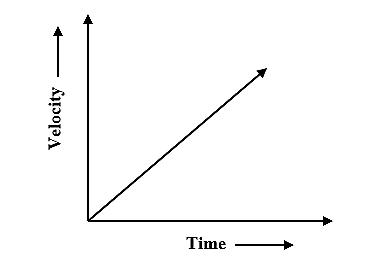# Uniform Acceleration Study Guide

What is Uniform Acceleration

We’ve all admired the concept of tossing an object from a great height and seeing it speed up till it lands. This crazy conduct by us incorporates a number of physical science principles. The object we toss falls effectively free, and despite the air resistance it encounters, it accelerates the entire way down. Let’s look at the concept of constant acceleration in more detail.

## Acceleration and Its Types

Any process in which the velocity varies is referred to as acceleration. Because velocity is both a speed and a direction, you can only accelerate in one of two ways: alter your speed or modify your direction—or both. Acceleration is a vector quantity that is described as the rate of change in velocity. The acceleration can be positive, negative, or zero depending upon the velocity and its direction.

Mainly Uniform acceleration, non-uniform acceleration, and average acceleration are the three types of accelerated motions.

Regardless of the time period length, a non-uniform accelerated motion item does not travel the very same distance in equal intervals of time.

The average acceleration during a certain interval is accompanied by a change in velocity for that period.

## Uniform Acceleration

We’re all aware of the concept of uniform motion: a body is said to be in uniform motion if it travels the same distance in the same amount of time. Now, uniformly accelerated motion means that an object or a body possesses __constant acceleration. __

In other words, uniform acceleration’s definition can be when an object moves in a single direction and its velocity changes with time or intervals and remains constant. A ball moving down a hill and a child tumbling down the slide are some of the common uniform acceleration examples.

There are three equations of uniformly accelerated motion that can be used are

• s= ut+1/2 a t2

• v= u+at

• And, v2= u2+2as

Where,

v – The final Velocity

u – The initial Velocity

a – Acceleration

s – The distance

t – The time interval

The velocity-time graph for uniform acceleration will be as-.### Characteristics of Uniformly Accelerated Motion

• Positive acceleration will arise if the direction of both acceleration and the change in velocity are the same.

• At the same time, negative acceleration, sometimes referred to as retardation or deceleration, will arise if the direction of acceleration and the change in velocity are different or in the opposite direction.

• Positive acceleration causes the object’s speed to either increase or decrease, while negative acceleration causes the object’s speed to slow down, which is why it is also referred to as retardation.

###Summary

• Any process in which the velocity varies is referred to as acceleration.
• Mainly, Uniform acceleration, non-uniform acceleration, and average acceleration are the three types of accelerated motions.
• The term uniform acceleration refers to a motion wherein an object travels in a straight line with an increase in velocity at equal intervals of time.

## FAQs

1. When will you say a body is in uniform acceleration?

A body is in uniform acceleration when it moves in a single direction, and its velocity does not change with time.

2. What is the velocity-time graph for uniform acceleration?

During uniform accelerated motion, the velocity-time graph is a straight line.

3. What is uniformly accelerated motion?

The term uniform acceleration refers to a motion wherein an object travels in a straight line with an increase in velocity at equal intervals of time.

We hope you enjoyed studying this lesson and learned something cool about Uniform Acceleration! Join our Discord community to get any questions you may have answered and to engage with other students just like you! Don’t forget to download our App to experience our fun VR classrooms – we promise, it makes studying much more fun!😎

]]>